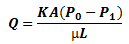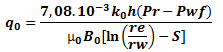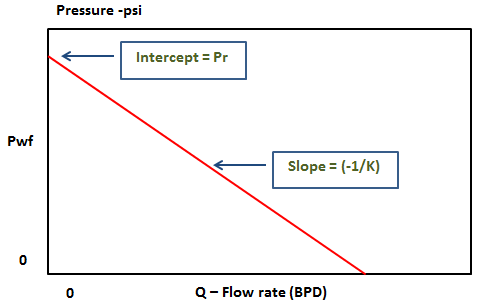# Well Inflow Performance

Well Inflow Performance represents the relationship between pressure and flow rate at the well face of an individual well. The scientist Darcy was the first studied extensively the relationship between pressure and flow rate. His experimental studies consist on creating a pressure differential across a porous media and measured the resulting flow rate.Darcy’s experiments result in what is known as “Darcy’s law”. For general flow through a porous media:The Darcy’s law can be written for any geometry, but radial flow geometry is the one of most interest to the production engineer dealing with single well issues. Considering radial flow geometry, Darcy’s law looks like:• q0= Flow rate
• h= Effective feet of pay
• Pr= Reservoir pressure
• Pwf= Wellbore pressure
• re= Drainage pressure
• B0= Formation volume factor
• k0= Effective permeability
• µ0=Average viscosity

### Productivity Index Model:

For a particular well, we can assume that h, Pr, Pwf, re, rw, B0, k0 and µ0 are constant. Thus replaced by K:

q0 = K (Pr – Pwf)

The constant K is known as Productivity index or simply “PI”. PI is often called J in some textbooks.

The productivity index (PI) is equal to the liquid flow rate divided by the well drawdown (Pr-Pwf).

[PI] = bbl/day/psi

q0 = PI (Pr – Pwf)

Pwf = (-1/PI) q0 + Pr

Graphically, it looks like this:The maximum flow rate occurs at the maximum drawdown (Pwf=0).

PI = qmax / (Pr – 0)

Then: qmax = Pr × PI

The following video shows more details about Productivity Index Inflow Performance:

Other inflow performance relationships exist in the literature (i.e. Vogel, Composite, and Fetkovich) and will be detailed in dedicated articles in the Inflow & Outflow Performance Category of this website.

### You May Also Like…1.Antonio Neto says: